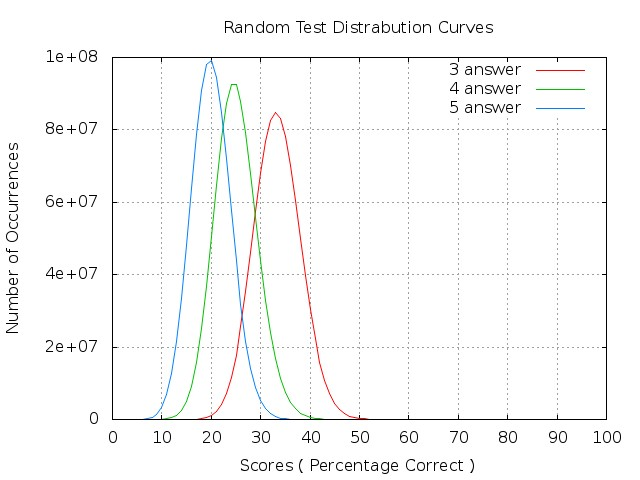# doom'd net

still mucking around with the ol'computer

# Can Someone Pass the SAT, or Anyother Filling the Bubble Type Test, by Randomly Answering Each Question?The What If? blog recently asked What if everyone who took the SAT guessed on every multiple-choice question? How many perfect scores would there be?

This got me thinking.  Since using the Monte Carlo Method is one of my favorite methods to solve problems, it seemed that this would be a perfect problem to solve.

I greatly simplified things from a SAT scoring system.  But I wrote a program that would create a random test key with a specified number of solutions, and then take a specified number of problems for a specified number of tests.

I ran this program with 3, 4, and 5 solution problems, with 100 problems per test, for 1 billion tests each ( just about the maximum my computer can reasonably handle ).  I used a simple scoring system where the number of right answers for each test was the percentage of the test ( hence the 100 questions ).

What I found was that the average was 33.33%, 25%, and 20% respectively.  No test, out of 3 billion runs had a perfect 100%.

Here are the Standard Distribution Curves:Here is a final thought.  I had a teacher point out that if as student gets a score that is the average +/- 1 standard deviation, they had to work for that score. If it’s a high score, no problem, but if it’s a low score, then the student had to actually work harder than just guessing to get a LOWER score!

Update as of 05/13/2015: The C program, scripts, and data files used to create this entry are now available on GitHub: https://github.com/lorddoomicus/tester.